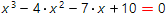# Solve cubic equation onlineCubic equationis equation of the form:

a∙x3 + b∙x2 + c∙x + d = 0

Presented online calculator solves cubic equations using Cardano formulae. However, in special cases (then one or more coefficients are equal to zero, or there is some dependence between coefficients, ect.) more simple solution is used.

Numbers, fractions and even parameters allowed as the coefficients input. The coefficient at x3 (the leading one) can't be zero. The equation entered into calculator in its natural form. To enter the decimal numbers, use point as the integer and decimal parts separator, for instance 1.45.

Cubic equations solver
Use decimal numbersCubic equation you want to solve: